Next: Application to Bayesian Noisy Up: Fast Algorithms for Bayesian Previous: FastICA as EM-Algorithm with

# Application to General ICA Algorithms

The procedure giving faster convergence derived in previous sections is a general approach and FastICA was seen to be a special case. Since the faster convergence was achieved by comparing the re-estimation step to Gaussian noise removal, the approach is valid for any situation where the general noisy ICA model holds with Gaussian noise and linear mixtures. It is not required that the E-step uses the approximation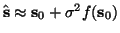; instead, it can be any method that can use s0 to compute. Denote this estimation by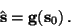Then it is always possible to replace the source with Gaussianized source s0G and obtain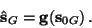Having estimated two sets of sources, we can apply any method whatsoever to estimate the mixing matrix using the newly estimated sources. This gives us two new estimates of the vectors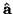and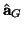of the mixing matrix. The final estimate is obtained as the normalized difference as above.

Harri Lappalainen
2000-03-09